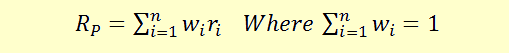# Expected Value of a Portfolio

We earlier learned about how to calculate the expected value, variance, and standard deviation of a single random variable or an asset.

Portfolio managers will have many assets in their portfolios in different proportions. The portfolio manager will have to therefore calculate the returns on the entire portfolio of assets. The returns on the portfolio are calculated as the weighted average of the returns on all the assets held in the portfolio.

Using the same properties, we can calculate the expected value (returns), variance and standard deviation of a portfolio.

##### Expected Value (Expected returns)

The formula for portfolio returns is presented below:w represents the weights of each asset, and r represents the returns on the assets. For example, if an asset constitutes 25% of the portfolio, its weight will be 0.25. Note that sum of all the asset weights will be equal to 1, as it will represent 100% of the investment. The returns here are single period returns with same periods for each asset’s returns.

Let’s take an example of a two asset portfolio to understand how portfolio returns are calculated. Let’s say that our portfolio comprises of two assets A and B and has the following details.

 Investment Returns A 25000 10% B 75000 6%

# Checkout our eBooks and Templates

eBooks and templates related to finance, R programming, Python, and Excel.
Visit Store
Get our Data Science for Finance Bundle for just $29$51. That's 43% OFF.
Get it for $51$29